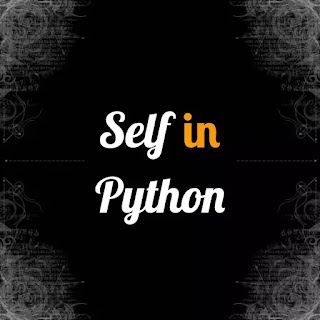# Self in python - codebugfree

In this section we learn about the uses or important of self in python programmer. Self is basic things or property of oops. OOPs full form is object oriented programing. Self is use in instance attributes and class attributes.Self in python.

## What is the use of Self in Python

We can access the class attribute by using self keyword . Because python class use self keyword but their to specific keyword like"@" to refer the python instance attributes. So in python we use self keyboard.

Note this point beginner programmer is confused in self.  At last time say that self means i am. If run a oops class than using self all attributes gives equal value to other objects.

We will se in the program to use of self and work in self in python programming.

``````class self_in_python():#class
def __init__(self):# init constructor with self
#down below instance variable
self.self_in_python_use = "This is of self"
self.why_self_use_python = "because it gives equality of all object"

self_python = self_in_python()#creating the object
print(self_python.self_in_python_use)
print(self_python.why_self_use_python)
self_gives_equal = self_in_python()# creating the another object
print(self_gives_equal.self_in_python_use)
print(self_gives_equal.why_self_use_python)``````

First we make the oops class with name self in python. making the init constructor. make the instance variable down below. creating the first object and print the instance variable. Creating the second object and print the instance variable.

INOUTPUT

``````This is of self
because it gives equality of all object
This is of self
because it gives equality of all object``````

It is a beginner practice problem and we see the advance problem using the self keyboard. Also use init constructor and instance variable. But it is use a difficult way.

``````class Self_in_python():#creating class
def __init__(self,what_use,why_use): # init constructor passing attributes to object
self.what_use = what_use
self.why_use = why_use
def print_self_info(self):
return f"this is answer of what use {self.what_use}"
def printing_self_use(self):
return f"this is the answer of why use {self.why_use}"
# first object
using_self_in_python = Self_in_python("we use because self makes program good and read ale","We use because it is easy to use")
print(using_self_in_python.print_self_info())
print(using_self_in_python.printing_self_use())
#second object
another_self_use = Self_in_python("i am use java not python","i am use alter native of self")
print(another_self_use.print_self_info())
print(another_self_use.printing_self_use())``````

It is harder than the first project. We first we make class. create the init constructor with passing attributes to the variable. Creating the function  to print the data from object. creating the first object and inside the () input the string. last print the string with the help of function.

Second object is create and input the sting inside the () and printing all string with the help of function. Ther function is return so down the object we use the print statements.

INOUTPUT

``````this is answer of what use we use because self makes program good and read ale
this is the answer of why use We use because it is easy to use
this is answer of what use i am use java not python
this is the answer of why use i am use alter native of self``````